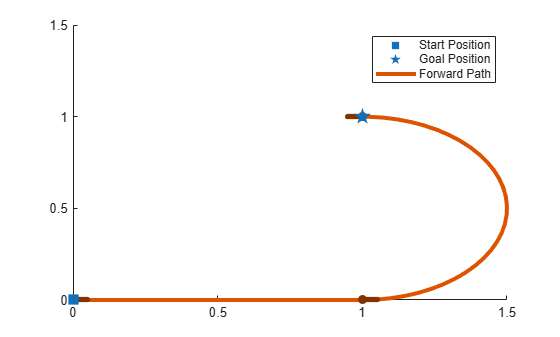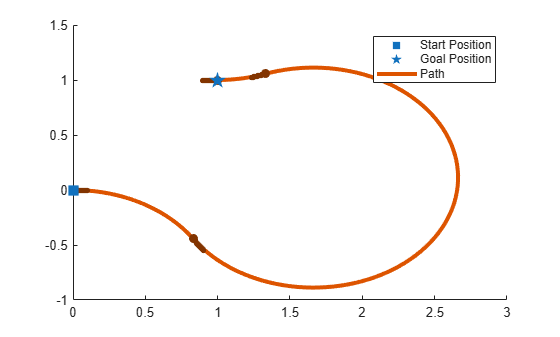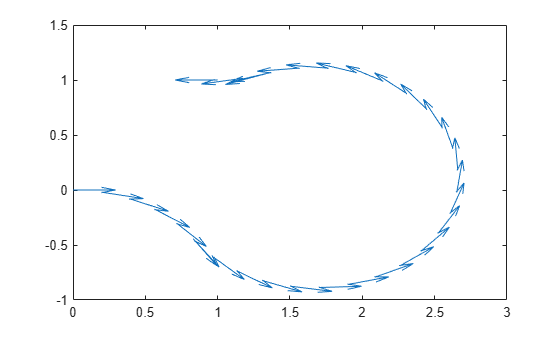# show

Visualize path segment

## Syntax

``show(pathSeg)``
``show(pathSeg,Name,Value)``

## Description

example

````show(pathSeg)` plots the path segment with start and goal positions and their headings.```
````show(pathSeg,Name,Value)` also specifies `Name,Value` pairs to control display settings.```

## Examples

collapse all

Create a `dubinsConnection` object.

`dubConnObj = dubinsConnection;`

Define start and goal poses as `[x y theta]` vectors.

```startPose = [0 0 0]; goalPose = [1 1 pi];```

Calculate a valid path segment to connect the poses.

`[pathSegObj, pathCosts] = connect(dubConnObj,startPose,goalPose);`

Show the generated path.

`show(pathSegObj{1})`Create a `reedsSheppConnection` object.

`reedsConnObj = reedsSheppConnection;`

Define start and goal poses as `[x y theta]` vectors.

```startPose = [0 0 0]; goalPose = [1 1 pi];```

Calculate a valid path segment to connect the poses.

`[pathSegObj,pathCosts] = connect(reedsConnObj,startPose,goalPose);`

Show the generated path. Notice the direction of the turns.

`show(pathSegObj{1})``pathSegObj{1}.MotionTypes`
```ans = 1x5 cell {'L'} {'R'} {'L'} {'N'} {'N'} ```
`pathSegObj{1}.MotionDirections`
```ans = 1×5 1 -1 1 1 1 ```

Disable this specific motion sequence in a new connection object. Reduce the `MinTurningRadius` if the robot is more maneuverable. Increase the reverse cost to reduce the likelihood of reverse directions being used. Connect the poses again to get a different path.

```reedsConnObj = reedsSheppConnection('DisabledPathTypes',{'LpRnLp'}); reedsConnObj.MinTurningRadius = 0.5; reedsConnObj.ReverseCost = 5; [pathSegObj,pathCosts] = connect(reedsConnObj,startPose,goalPose); pathSegObj{1}.MotionTypes```
```ans = 1x5 cell {'L'} {'S'} {'L'} {'N'} {'N'} ```
```show(pathSegObj{1}) xlim([0 1.5]) ylim([0 1.5])```Create a `dubinsConnection` object.

`dubConnObj = dubinsConnection;`

Define start and goal poses as `[x y theta]` vectors.

```startPose = [0 0 0]; goalPose = [1 1 pi];```

Calculate a valid path segment to connect the poses.

`[pathSegObj,pathCosts] = connect(dubConnObj,startPose,goalPose);`

Show the generated path.

`show(pathSegObj{1})`Interpolate poses along the path. Get a pose every 0.2 meters, including the transitions between turns.

```length = pathSegObj{1}.Length; poses = interpolate(pathSegObj{1},0:0.2:length)```
```poses = 32×3 0 0 0 0.1987 -0.0199 6.0832 0.3894 -0.0789 5.8832 0.5646 -0.1747 5.6832 0.7174 -0.3033 5.4832 0.8309 -0.4436 5.3024 0.8418 -0.4595 5.3216 0.9718 -0.6110 5.5216 1.1293 -0.7337 5.7216 1.3081 -0.8226 5.9216 ⋮ ```

Use the `quiver` function to plot these poses.

`quiver(poses(:,1),poses(:,2),cos(poses(:,3)),sin(poses(:,3)),0.5)`## Input Arguments

collapse all

### Name-Value Arguments

Specify optional pairs of arguments as `Name1=Value1,...,NameN=ValueN`, where `Name` is the argument name and `Value` is the corresponding value. Name-value arguments must appear after other arguments, but the order of the pairs does not matter.

Before R2021a, use commas to separate each name and value, and enclose `Name` in quotes.

Example: `'Headings',{'transitions'}`

Axes to plot path onto, specified as an `Axes` handle.

Heading angles to display, specified as a cell array of character vector or string scalars. Options are any combination of `'start'`,`'goal'`, and `'transitions'`. To disable all heading displays, specify `{''}`.

Positions to display, specified as `'both'`, `'start'`, `'goal'`, or `'none'`. The start position is marked with green, and the goal position is marked with red.

Length of heading, specified as positive numeric scalar. By default the value is calculated according to the `x`- and `y`-axis limits of the plot.

Data Types: `double`

## Version History

Introduced in R2019b